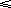#### You may also like### Double Digit### Whole Numbers Only

Can you work out how many of each kind of pencil this student bought?### The Patent Solution

A combination mechanism for a safe comprises thirty-two tumblers numbered from one to thirty-two in such a way that the numbers in each wheel total 132... Could you open the safe?

# Not a Polite Question

##### Age 11 to 14 ShortChallenge Level

If her age is 10 t + u (where t is the tens digit and u is the units digit) then reversing the digits gives 10 u + t. and the sum is 11 t + 11 u, which is a multiple of 11. We know this has to be a square number. Ong Xing Cong from Singapore sent in the following solution.

11 t + 11 u = 11 x 11 = 121

t + u = 11

65 - 56 = 3 x 3

She is 65 years old.

The best solutions do not need trial and improvement methods and they show that the answer or answers found are the only possible answers. Knowing the digits add up to 11 ( t + u = 11), you can also use (10 t + u ) - (10 u + t ) = 9 t - 9 u = 9( t - u ) As this is also a square number you know ( t - u ) is either 1, 4, or 9. The solutions for 4 and 9 don't give whole number values for t in 0t9.

You can find more short problems, arranged by curriculum topic, in our short problems collection.